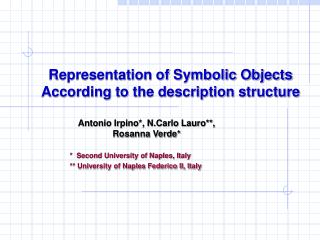DownloadDownload PresentationRepresentation of Symbolic Objects According to the description structure

# Representation of Symbolic Objects According to the description structure

Télécharger la présentation## Representation of Symbolic Objects According to the description structure

- - - - - - - - - - - - - - - - - - - - - - - - - - - E N D - - - - - - - - - - - - - - - - - - - - - - - - - - -
##### Presentation Transcript

1. Representation of Symbolic Objects According to the description structure Antonio Irpino*, N.Carlo Lauro**, Rosanna Verde* * Second University of Naples, Italy ** University of Naples Federico II, Italy

2. Problem • The results of the factorial analysis as well as the symbolic objects visualization depend on the nature and structure of symbolic descriptors • Aims • To transform symbolic data in input in order to: • homogenize the nature of the different descriptors; • study (non-linear) relationships among descriptors; • Find the most suitable kind of visualizatiob of the symbolic objects (by connected shapes)

3. Transformation of the SO descriptors in Factorial Analysis

4. GCA analysis allows to perform a reduction of dimensionality of the original descriptors space. The different kind of descriptors in input are transformed in multi-categorial descriptors with associated weights

5. Coherent Transformation of descriptors(Homeomorphism) • From a geometrical point of view – let give a topology on a space, the topology defined on the tranformed space have to be an homeomorphism. • The transformation function have to be biettive and bicontinue. • From a statistical point of view - the transformation do not allow to lose information related to the internal variability of the objects. • Furthermore we have to take into account the metric of spaces. • For istance, the classical categorization of a real variable is not a homeomorphic transformation

6. Transformation of a single numerical variable into a multicategorial modal one k R Euclidean metric Y1 = k L M 1 Metric : c2 1 F(k)=(L(k), M(k), H(k)) Lk+Mk+Hk=1 Lk,Mk,Hk[0,1] 0 1 H f:(S,m)(S’,m’) biettive and bicontinue (f,f -1are continue) where: S=[min, Max]  R , min < MAX S’=(L, M, H) R3 L,M,H[0,1] L+M+H=1; m=c2

7. Representation of symbolic assertion in the original space where the descriptors are interval variables

8. Example of tranformation of an interval ina multi-categorial with associated weights R u l Euclidean metric Min<l<k<u<Max L M L(k), M(k), H(k) L(k)+M(k)+H(k) =1 X2 metric H It is worth to note that it needs to codify with respect to others points than the min and Max in order to keep an homeomorfism: (0,1,(0)

9. Example of tranformation of an interval in a multi-categorial with associated weights using semilinear B-spline functions Euclidean metric R u l Min<l<k<u<Max L M L(k), M(k), H(k) L(k)+M(k)+H(k) =1 X2 metric H

10. Example of tranformation of an interval in a multicategorial weighted value using semilinear B-spline function(2) Euclidean metric R u l Min<l<k<u<Max ML L(k), ML(k), MH(k),H(k) k+ML+MH+H=1 H c2 metric L MH

11. Transformation of multinominal variable(1) What is the topology which take into accout the variability? {Red, Green, Blue} is not a metric space Red Red Green 1 Green 1 1 1 c2 metric Two Points A segment Blue Blue 0 0 1 1

12. Transforming multinominal variable(2) 1 2 3 Red Green Blue

13. Transformation multinominal variable(3) Multicategorial modal variable with weights at interval Red Green Blue

14. Example of representation of a symbolic assertion in the original description space(An interval variable combined with a Multinominal Variable) (1)

15. Example of representation of Symbolic Assertion in the original description space(An interval variable conbined with a Multinominal Variable) (2) The representation is no convex as MCAR but connected

16. Example of representation of a symbolic assertion Blue Green Red

17. Effects of the descriptors transformation on the visualization on factorial planes • In factorial analysis are performed orthogonal projections onto factorial subspaces. • A factorial subspace is a linear combination of the symbolic descriptors • This means that: the space spanned by factorial variables is an affine transformation of the originary space • An affine trasformation is invariant with respect to linear properties of the originary space i.e.: a linear projection of a convex shape is a convex shape and so on.

18. Choice of the most suitable SO visualization(1) • A linear projection of a convex shape is a convex shape MCAR Originary space Factorial plane Convex hull of the vertices is the best visualization shape (i.e. no-overfitting)

19. Choice of the most suitable SO visualization (2) A linear projection of a connected shape is a connected shape Convex hull of the vertices is better than MCAR but it presents an overfitting CH MCAR Originary space Factorial plane

20. Descriptors’ space with rules When there are dependences rules, then the descriptor space loses the convexity properties R: If y1>h then Y2<k Y2 Incoherent sub space induced by the dependence rule k Y1 h

21. Analysis of non-linear relationships between variables • By tranforming interval variables into categorical modal ones - by means of semilinear B-splines functions - it is possible to study non linear relationships among variables. • It allows to study relationships between categories of two or more variables which represent an High Medium or Low level of them.

22. Open problems • A new kind of symbolic variable needs • Multicategorial modal having weights at interval • What is the topological structure and the properties of this kind of geometrical space? • New visualization shapes need in order to solve overfitting problem. • Symbolic interpretation of the shapes of SO representation